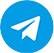# TOP 10 SAP FICO – Asset Accounting Interview Questions

0
19241

Below are some important questions on SAP FICO Asset Accounting for beginners and professionals. Some of these are asked in TOP MNC company interviews.

1. What is Chart of Depreciation ?
The Chart of Depreciation is a list of Depreciation  Areas arranges according to business and legal requirement.
Chart of Depreciation is used in order to manage various legal requirements for the depreciation and valuation of assets. Each company code is allocated to one chart of depreciation.

2.What do you mean by Depreciation Area ?
Methods of calculation of depreciation. An area showing a valuation of fixed assets for a particular purpose. For example Book depreciation, Income Tax Depreciation, Costing depreciation.

3. What is Account Determination ?
One of the most important functions of the Asset class to be establish the connection between the Asset master record and the corresponding accounts in the general ledger in Financial accounting. This connection is created by the account determination key is asset class.

4. What is screen Layout ?
Design of the records and design of the documents.

5. What is Asset Class ?
The Asset class is the main criteria for classifying assets. Every asset must be assigned to any one class.The asset class is nothing but a group of asset. For example of Asset classes are Plant & Machinery, Furniture & Fixtures, Computer etc…

6. What is Depreciation key ?
A key for calculating depreciation amounts.There is a direct link between asset and company code.Which we link between the depreciation key and master record.

7, What is an asset master ? What does it control for sub asset master ?
An asset master represents the master record and information about a particular asset. According to the screen layout of asset master, it controls the following sub assets

• Sub assets master number assignment
• Assignment of depreciation key
• Determination of life asset
• determination of assignment of group asset.

8. How many depreciation areas can be defined for a Company Code ?
Depreciation areas are not defined for company codes rather depreciation areas are defined for a chart of depreciation, A maximum f 99 depreciation areas cab be maintained for a Chart of Depreciation.

9. What are the types of Depreciation methods ?
Base method

• Decline value method
• Maximum method
• Multi level method
• Period control method.

10. Is it possible to create an asset class automatically ?
Yes, There are two ways 1. Create an asset class with reference to an existing asset class
2.Generate an asset class through the asset class transaction code(ANKL).

11. How many ways can you create the asset master record ?

• With reference to an asset
• Using the number functionality for similar assets.
• Through an asset class(AS01).

12. What is the difference between COA and COD ?
The chart of Accounts is the index of G/L accounts.The Chart of Account can be global,country specific or industry specific based on the need of business. The COD is the index of depreciation areas.The COD is only country specific.

13. What are the segments in asset master ?
Master data segment   and   Depreciation segment.SAPLearners.com is now on Telegram. Click here to join our channel and stay updated with the latest tutorials and updates.